Maths-
General
Easy

Question

# A function y = f (x) satisfies the condition f '(x) sin x + f (x) cos x = 1, f (x) being bounded when x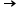0. If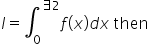## The correct answer is: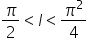### Given:-A function y=f(x) satisfies the condition f′(x)sinx+f(x)cosx=1,f(x) being bounded when x→0we need to find I if I=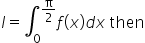.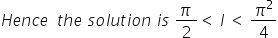#### With Turito Foundation.#### Get an Expert Advice From Turito.